Test: Electrical Machines- 8

# Test: Electrical Machines- 8

Test Description

## 10 Questions MCQ Test GATE Electrical Engineering (EE) 2023 Mock Test Series | Test: Electrical Machines- 8

Test: Electrical Machines- 8 for Electrical Engineering (EE) 2023 is part of GATE Electrical Engineering (EE) 2023 Mock Test Series preparation. The Test: Electrical Machines- 8 questions and answers have been prepared according to the Electrical Engineering (EE) exam syllabus.The Test: Electrical Machines- 8 MCQs are made for Electrical Engineering (EE) 2023 Exam. Find important definitions, questions, notes, meanings, examples, exercises, MCQs and online tests for Test: Electrical Machines- 8 below.
Solutions of Test: Electrical Machines- 8 questions in English are available as part of our GATE Electrical Engineering (EE) 2023 Mock Test Series for Electrical Engineering (EE) & Test: Electrical Machines- 8 solutions in Hindi for GATE Electrical Engineering (EE) 2023 Mock Test Series course. Download more important topics, notes, lectures and mock test series for Electrical Engineering (EE) Exam by signing up for free. Attempt Test: Electrical Machines- 8 | 10 questions in 30 minutes | Mock test for Electrical Engineering (EE) preparation | Free important questions MCQ to study GATE Electrical Engineering (EE) 2023 Mock Test Series for Electrical Engineering (EE) Exam | Download free PDF with solutions
 1 Crore+ students have signed up on EduRev. Have you?
Test: Electrical Machines- 8 - Question 1

### A 3-phase synchronous motor of 10 KW at 1.3 kV has a synchronous reactance of 10 Ω/phase. The efficiency of the machine is 75%. Find input power for the motor.

Detailed Solution for Test: Electrical Machines- 8 - Question 1

Given that, output to the motor (Pout) = 10 kW
Efficiency (η) = 0.75
Motor line current is minimum at cos ϕ = 1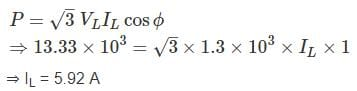Input to motor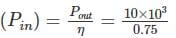= 13.33 kW

Test: Electrical Machines- 8 - Question 2

### A synchronous motor with negligible armature resistance runs at a load angle of 20° at the rated frequency. If supply frequency is increased by 10%, keeping the other parameters constant, the new load angle will be

Detailed Solution for Test: Electrical Machines- 8 - Question 2

Power delivered by the synchronous motor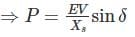From data, other parameters such as P, E and V are constant.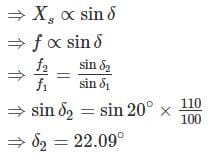*Answer can only contain numeric values
Test: Electrical Machines- 8 - Question 3

### The per phase circuit equivalent of a synchronous generator feeding a synchronous motor through a transmission line is shown in fig. Each machine is rated 10 kVA, 400V, 50 Hz. The motor is driving a load of 8 kW. The field current of the two machine are so adjusted that the motor terminal voltage is 400V and its pf is 0.8 leading. Find the minimum value of Em (in P.U) for the machine to remain in synchronism.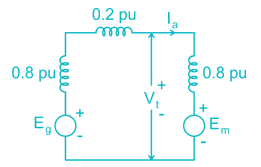Detailed Solution for Test: Electrical Machines- 8 - Question 3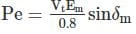For maximum Em, δm = 90° limit of stability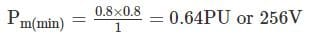Test: Electrical Machines- 8 - Question 4

The rotor of a 50 Hz, 4 poles, 3ϕ synchronous motor is directly coupled to the rotor of a 50 Hz, 6 poles induction motor. If the stators of both machines are given balanced 3ϕ, 50 Hz supplies of rated voltage values. The possible induced frequencies in the rotor of induction motor are-

Detailed Solution for Test: Electrical Machines- 8 - Question 4

We know that, N = 120f/P
Speed of synchronous motor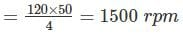Speed of induction motor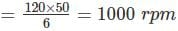Given that both the rotors are directly coupled.
If rotating field is in the opposite direction to that of rotor speed.
Speed = 1500 + 1000 = 2500 rpm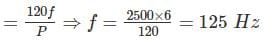If rotating field is in the same direction to that of rotor speed,
Speed = 1500 – 1000 = 500 rpm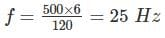*Answer can only contain numeric values
Test: Electrical Machines- 8 - Question 5

A cylindrical rotor synchronous motor is used to improve the power factor of a load 5 MW at a power factor of 0.6 lag. The motor is required to meet an additional demand of 0.2 MW and raise the overall power factor to 0.8 (lag). If the efficiency of the motor is 75%. The power factor of the motor is – (up to two decimal places)

Detailed Solution for Test: Electrical Machines- 8 - Question 5

P1 = 5 MW, cos ϕ1 = 0.6
Q1 = P1 tan ϕ
= 5 × tan (cos-1 (0.6)) = 6.67 MVAR
Required motor output = 0.2 MW
Efficiency = 0.75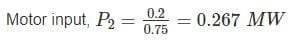Total load = 5 + 0.267 = 5.267 MW
New power factor cos ϕ = 0.8 lag.
Reactive power, Q = P tan ϕ
= 5.267 × tan (cos-1 (0.8))
= 3.95 MVAR
MVA supplied by motor, Q2 = Q1 – Q = 6.67 – 3.95 = 2.72 MVAR
Q2 = P2 tan ϕ2
⇒ 2.72 = 0.267 tanϕ2
⇒ ϕ2 = 84.39°
Cosϕ2 = 0.098

*Answer can only contain numeric values
Test: Electrical Machines- 8 - Question 6

A 60 MVA, 11 kV, 50 Hz, Y connected, 3 phase salient pole synchronous motor has direct axis synchronous reactance Xd = 1.8 Ω and quadrature axis synchronous reactance Xq = 1.2 Ω. If the armature resistance is negligible and full load power factor is 0.8 log. The power angle is-

Detailed Solution for Test: Electrical Machines- 8 - Question 6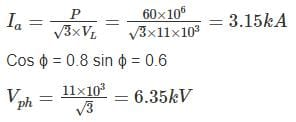For logging load in salient pole motor,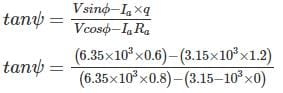ψ = 0.338°
δ + ψ = 3.338°
⇒ δ = 0.338° - 36.86° = -36.52°

*Answer can only contain numeric values
Test: Electrical Machines- 8 - Question 7

A 3-phase synchronous motor of 10 KW at 1.3 kV has synchronous reactance of 10 Ω/phase. The efficiency of the machine is 75%. The induced emf (per phase) corresponding to minimum current for full load condition is ____ (in V). Neglect armature resistance.

Detailed Solution for Test: Electrical Machines- 8 - Question 7

Given that, output to motor (Pout) = 10 kW
Efficiency (η) = 0.75
Motor line current is minimum at cos ϕ = 1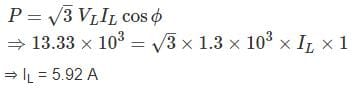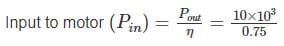= 13.33 kW
Impedance drop = I × synchronous impedance
= 5.92 × 10 = 59.20 V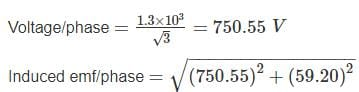= 752.89 V

*Answer can only contain numeric values
Test: Electrical Machines- 8 - Question 8

A 400 V, 3ϕ delta connected synchronous motor runs at rated voltage and with an excitation emf of 500 V. Its winding and iron losses are 1800 W and synchronous impedance per phase is (0.7 + 3j) Ω. Calculate the shaft power output in kW

Detailed Solution for Test: Electrical Machines- 8 - Question 8

Zs = (0.7 + 3j) = 3.08 ∠76.87
Vt = 400 V, Ef = 500 V
Maximum out put power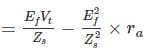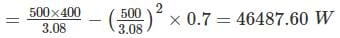Shaft power = [3 × 46.49 – 1.8]kW = 137.66 kW

*Answer can only contain numeric values
Test: Electrical Machines- 8 - Question 9

The maximum power delivered by 1500 kW, three phase star connected 4 kV, 48 pole 50 Hz synchronous motor, with synchronous reactance of 4 Ω per phase and unity power factor is___(in MW)

Detailed Solution for Test: Electrical Machines- 8 - Question 9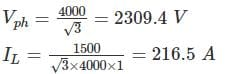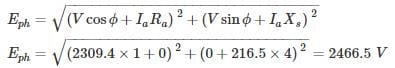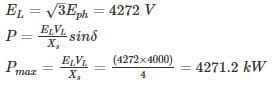Test: Electrical Machines- 8 - Question 10

A 2300 V, 400 h.p, 60 Hz, 8 pole Y connected synchronous motor has a rated power factor of 0.85 leading. At full load, the efficiency 85%. The armature resistance is 0.4, and the synchronous reactance is 4.4 Ω. Find the Ea for this machine when it is operating at full load.

Detailed Solution for Test: Electrical Machines- 8 - Question 10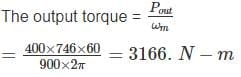The input power is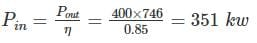The armature current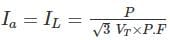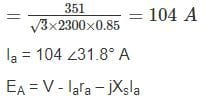= 1328 ∠0° - 0.4 × 104∠31.8° - j × 4.4 × 104∠31.8°

Ea = 1588 ∠-15° V

## GATE Electrical Engineering (EE) 2023 Mock Test Series

22 docs|274 tests
 Use Code STAYHOME200 and get INR 200 additional OFF Use Coupon Code
Information about Test: Electrical Machines- 8 Page
In this test you can find the Exam questions for Test: Electrical Machines- 8 solved & explained in the simplest way possible. Besides giving Questions and answers for Test: Electrical Machines- 8, EduRev gives you an ample number of Online tests for practice

## GATE Electrical Engineering (EE) 2023 Mock Test Series

22 docs|274 tests